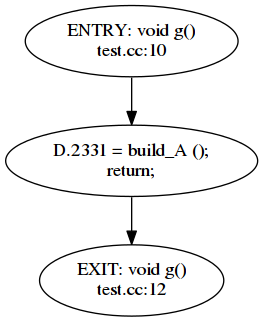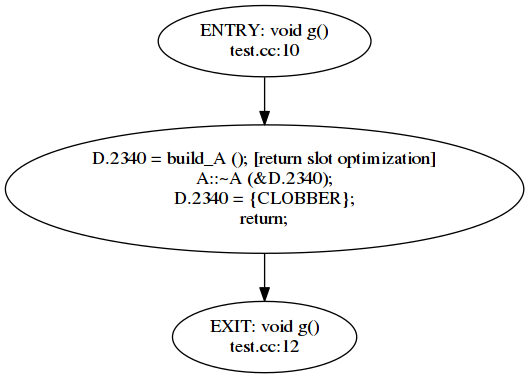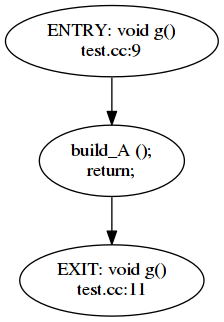In the last chapter, we extended our plugin so it was possible to visualize the control flow graph of a function. In this chapter we will reach our goal to warn unused results of function calls.

## GIMPLE

In the previous part, we saw that compilers may use several intermediate representations during the lowering process. Our pass is a GIMPLE pass so it uses GIMPLE as the intermediate representation. If you check the graphs in the previous part, you will see that there is code in the nodes of the graph: this code is represented using GIMPLE. GIMPLE is a simplified representation that, except for function calls, has at most three operands (actually it can have four operands because of a special case of the conditional operator, but nevermind). This means that a statement like `a = b * c + d;` becomes the sequence `T1 = b * c; a = T1 + d;`. If you check the control flow graph drawn, you will see that these temporaries in GCC are usually of the form `D.nnnn`, but their exact name is actually unimportant (as long as it is used correctly into the simplified code, of course).

GIMPLE represents the program code using a set of GIMPLE statements. Each GIMPLE statement has a kind which denotes what it represent. Each kind of GIMPLE has a different number of operands. In this post will focus on two statements: `GIMPLE_ASSIGN` and `GIMPLE_CALL`.

A statement of kind `GIMPLE_ASSIGNMENT` is used to represent statements like the following ones

Here `⊕` represents a binary operation like addition, subtraction, multiplication, etc. The second form `a = b;` corresponds to the copy of a value. The operands `b` and `c` are called the right hand side (RHS) of the assignment, while the operand `a` is called the left hand side (LHS) of the assignment.

A statement of kind `GIMPLE_CALL` represent calls for two forms as well.

Where `F` is something that can be called: the name of a function or a variable that denotes a pointer to function. The operand `a` is the left hand side of the call, and may be omitted for the cases where the result is unused. Operands `x0`, `x1`, ..., `xN` are the arguments of the function call. It is possible to have zero arguments in a call of the form `a = F()` or `F()`. In the second form, either the function does not return anything (i.e. is void in C/C++) or its value is unused.

## Control flow graph of a call with unused results

Let's take again our phase from the previous chapter to draw the associated graph to the code we used to motivate this series of posts. Let me repeat it below.

Its associated CFG looks like this.Besides the ENTRY and EXIT, there is only a single basic block in function `g`. It contains two statements: a `GIMPLE_CALL` followed by another statement that simply does `return` (its kind is `GIMPLE_RETURN` but we will not care about it now). As you can see, the left hand side of the GIMPLE_CALL is still there.

### A class with user-declared destructor

Before we continue, let's make two more tests to better understand what is going on. First add a user-declared destructor to class `A`, the rest of the code is left untouched.

The control flow graph looks like this.This is much more complicated, the compiler must call the destructor of A with the temporary created (it also tags the call to build_A as using return value optimization but we do not care about this now). The statement with that `CLOBBER` thing is the way that GCC uses to know that the value of the variable is undefined at this point, this statement is actually a `GIMPLE_ASSIGN`.

### The function returns a non-class type

Now, remove the user-declared destructor but let's change function `build_A` to return a pointer to `A` (rather than a `A`), the rest of the code is left untouched.

Now the control flow graph looks like this.Hey! Where did the `lhs` of the function call go?

Well, the fun fact is that GCC uses the absence of the left hand side of a function call marked as `warn_unused_result` to determine if the warning must be emitted or not. You can verify that if you add `__attribute__((warn_unused_result))` at the beginning of the declaration of `build_A`: the warning is emitted for the last of three cases. Note that for the second case (where the destructor must be called) it is arguable if we want a diagnostic, at the moment let's assume we do not want it. So we want a diagnostic for the first case: when the destructor of a class is trivial and implies no code.

### Making sure the result is not used

While we may tolerate false negatives (i.e. not emitting a warning where it may make sense to emit it) we cannot accept false positives (the warning diagnoses something that does not happen). This means that we have to somehow be sure that the result is actually not used. Note that the left hand side of the call will not be a variable created by the user but a temporary variable created by the compiler. GCC calls them artificial declarations. To make sure that the temporary variable is not used at all (just written) we will have to verify all the statements in our function. We can do that by traversing the basic blocks. To simplify the process, we will structure it in two steps. The first step will gather the artificial variables that are a left hand side of a function call and put them in a set, but it may happen that the variable is actually used, so when this happens, it will be removed from the set. The second step, will traverse the code again and emit a diagnostic for all the places where the function returns an unused value and the function is marked with the attribute `warn_unused_result`. This strategy can be improved but as a start is probably enough.

Let's start laying out this strategy, we can begin with the code from part 2 (though this time I renamed the class from `my_first_pass` to `warn_unused_result_cxx`).

After the usual `pass_data` structure we find the actual definition of our pass. Now the execute method (lines 62 to 69) basically performs the two steps that I mentioned above: first we gather unused left hand sides (line 65) and then we will use this information to emit the warning (line 66).

Let's see the implementation of `gather_unused_lhs`

This seems a lot of code but basically we just traverse each basic block of the function (line 122). For each basic block we iterate each of its statements, using a GIMPLE statement iterator (`gimple_stmt_iterator`). Each GIMPLE statement (`gimple_stmt`) is then obtained from the iterator (line 127). As we mentioned above, there are several kinds of GIMPLE statements, so we will have to process them case by case. The kind of the GIMPLE statement is called in GCC lingo the GIMPLE code, so we get the gimple code and jump to the appropiate case (lines 129, 131 and 145).

When we find a function call (i.e. a `GIMPLE_CALL`) we will want to check if its left hand side is actually a temporary variable introduced by the compiler. If it is we will keep it in the set `potential_unused_lhs` (lines 119 and 134). We call this set, potential because at this point we are not 100% sure that the variable is actually unused. Function `insert_potentially_unused_lhs` does this check and if it succeeds it inserts the left hand side into `potential_unused_lhs_set`. We will see below its implementation.

If a potentially unused left hand side happens to be used later, we will have to remove it from the potentially unused set, because now it is not potentially unused: it is actually used! The variable may be used in any operand, so we will call `erase_if_used_lhs` for every operand. If the operand happens to be a previously potentially unused, we will remove it from the set. We will see below its implementation.

For function calls, this function has to be called for each argument of the function call (lines 136 to 141). For assignment operations (lines 147 to 159) we will call this function for every operand of the assignment: the left hand side and its (up to three) right hand sides.

The second step of our process involves emitting the warning. We do this in the function warn_unused_result_lhs.

You should identify a pretty similar structure, but in this case we only care about function calls, so is there only a case, all other GIMPLE statements are ignored. For a function call we get its left hand side (line 189) and verify if it is in the set of (actually) `unused_lhs`. This set has been computed in the first step of our process, and now all the potentially unused are actually unused.

The next lines of code are deliberately identical to the lines of code found in a real pass of GCC itself. That pass is the one responsible for emitting the diagnostic for `warn_unused_result`. We just use the same code: we get the function declaration (`fdecl`, line 193) from the function call and its type (`ftype`, line 194). We then make sure that we have to emit the diagnostic, by querying the type if it has the attribute `warn_unused_result` (line 196). If it does, then we emit the warning. The code in GCC verifies that the function declaration exists. This is so because this attribute could potentially be attached to pointer functions (and there is not a function for them). The diagnostic is emitted calling the GCC function `warning_at`, which requires the location of the statement (computed in line 198), a flag for the option (OPT_Wunused_result is the variable related to the GCC command-line parameter `-Wunused-result`, which happens to be enabled by default), and the message of the diagnostic (lines 200 to 208).

Now we can proceed to see the implementation of `insert_potentially_unused_lhs` and `erase_if_used_lhs`.

You will have already noticed but, `potential_unused_lhs` is a set of `tree`s. What is a `tree`? Well, for historical reasons probably (or maybe by design) there is a fundamental `tree` type that is used for almost anything inside GCC. It is structure that can hold many things: declarations, statements, expressions, types, etc. GIMPLE statements are not `tree`s but their operands are. So when we request the left hand side of a GIMPLE_CALL we get a tree. This tree type is actually another representation named in GCC as GENERIC. Due to the simplified nature of GIMPLE, though, tree operands are usually rather simple and do not carry a lot of complexity.

If our left hand side (recall, a `tree`) is NULL we do not have to do anything (line 81). Similar to the GIMPLE code, trees also have a code that is retrieved using the macro `TREE_CODE` (these macros are a witness of the story of GCC, initially written in C, I assume that they will be eventually replaced by C++ inline functions). If the code of the left hand side is a `VAR_DECL`, and this means it is a variable, we will verify, using the macro `DECL_ARTIFICIAL`, if it is an artificial variable created by the compiler (lines 84 and 85). If all these checks succeed, we will insert the tree into our `potential_unused_lhs` set (line 87).

In this function we will remove the variable declaration from the potential_unused_lhs set if it happens to be used. A trivial case is when the verified operand is an artificial VAR_DECL and happens to be in the `potential_unused_lhs` set. If it is we remove it from the set (note that for this case we could have just done `potential_unused_lhs.erase(t)` without having to check if anything about `t`, I wrote it this way for the sake of the exposition).

The second case, about COMPONENT_REF is there to solve this case.

In GIMPLE this will look like

When examining the function arguments of the call to some_function, we would not realize that `T1` is actually used because the argument is `T1.addr` (not as simple as `T1`). In this case we just call ourselves recursively with the left hand side of the "." (line 107). The left hand side can be retrieved using the macro `TREE_OPERAND(tree, child_number)`. In our case, the left hand side is found in the first children (counting from zero), thus `TREE_OPERAND(t, 0)`.

## Testing our plugin

Let's first change the check goal in the Makefile.

Our `test.cc` will have the following code.

Now we are ready to test it. Only two diagnostics should appear.

Woohoo!!

### A minor bug remains

If we add the following function at the end of our `test.cc`

it is diagnosed

This happens because we are not handling `GIMPLE_RETURN` in `gather_unused_lhs`. There is a missing case.

We simply call erase_if_used_lhs to the returned expression of this `GIMPLE_RETURN`. The `as_a<greturn*>` is just a cast required for these nodes (why such castings are not required for other nodes, I do not know, probably due to historical reasons again).

Woohoo!!

Well, it's been a long trip but now we have got a glimpse of writing GCC plugins and the GCC internals. You can find lots of information about the GCC internals in the GCC Internals Manual. Unfortunately it is not always up-to-date, so you will still have to check the code of GCC, but you already have it from the part 1, just check the files found in the `gcc` subdir inside `gcc-5.2.0`. You will definitely want to use ctags or a similar tool when reading the GCC code.

You can see the full code for this post here.